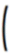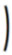##### Scaled

Each data sample requires two bytes of storage. You can therefore calculate the total disk storage necessary for each trend by using the following formula:

 Bytes necessary for each trend = 464 x No. Files + 176 +File Period (secs) x (No. Files) x 2Sample Period (secs)

For example, if a trend record produces one sample every ten seconds for one week, and you are using five data files (five weeks), the number of bytes necessary is:

 Bytes necessary = 464 x 5 + 176 +(7 x 24 x 60 x 60) x 5 x 210 Bytes necessary = 607,296 bytes

See Also